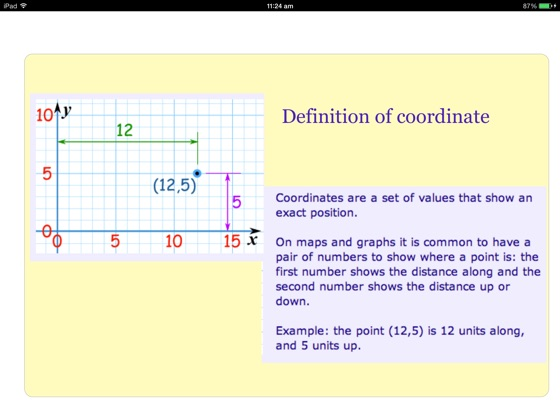## Publisher Description

Tidels Math Book Grade 5 focuses on Probability, Statistics, Pattern & Sequences, Consumer Math, Problem Solving, Number Theory & Mixed Operation, Time, Algebra, Number Theory, Fraction & Mixed Numbers. This e-book includes a collection of 200 math problems in the 12 categories. Students aged above 10 years can enjoy doing the problems.

Tidels brings you Math learning Workbook for your children to learn Fifth Grade Math Lessons.  The Complete Book of Math provides 200 pages of fun exercises for students in Grade 5 that teach students key lessons in math.

Learning the fundamentals of mathematics during the early years is crucial. Math Book helps your children in a  great way to practice the basics of mathematics. When children genuinely understand the basics of mathematics, they will have the confidence and capability to appreciate more advanced mathematics. Six to Eight year children will love these colorful and imaginative book. Our Math books are intended to enhance your child's skills and introduce new concepts in a fun-filled  and stress-free manner.

•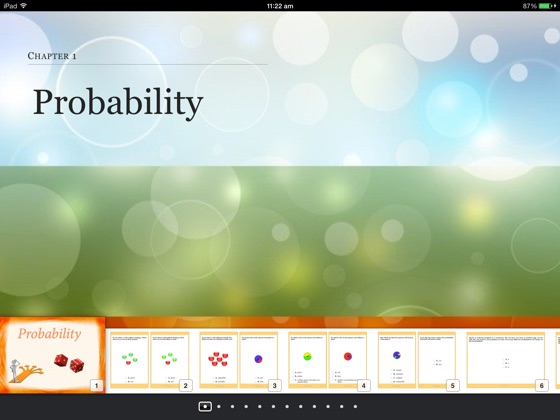•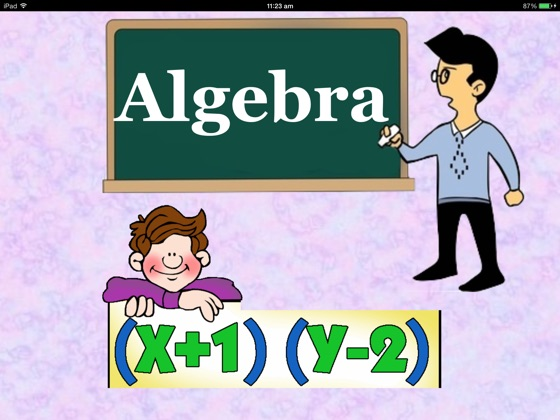•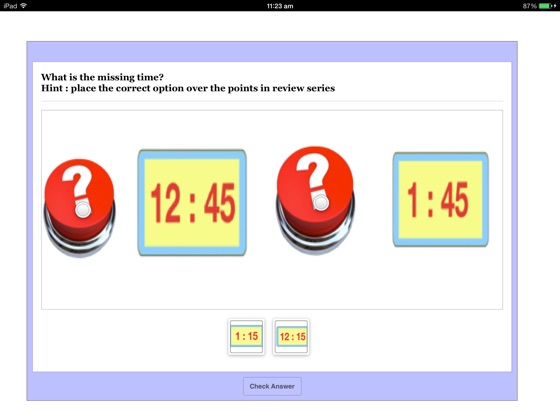•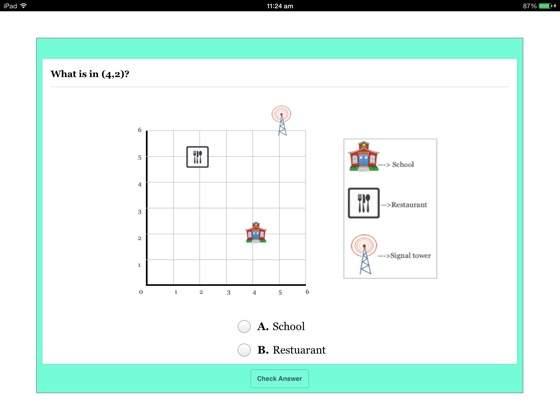•